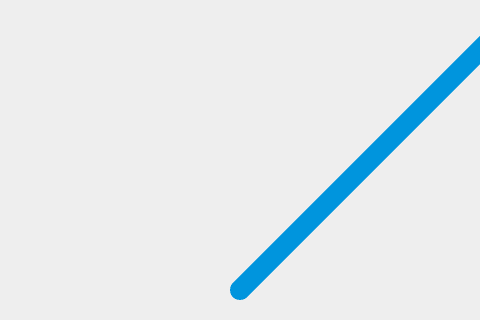# 讓球移動

## 定義一個繪製用的迴圈

js

``````function draw() {
// drawing code
}
setInterval(draw, 10);
``````

js

``````ctx.beginPath();
ctx.arc(50, 50, 10, 0, Math.PI * 2);
ctx.fillStyle = "#0095DD";
ctx.fill();
ctx.closePath();
``````

## 讓球動起來

js

``````var x = canvas.width / 2;
var y = canvas.height - 30;
``````

js

``````function draw() {
ctx.beginPath();
ctx.arc(x, y, 10, 0, Math.PI * 2);
ctx.fillStyle = "#0095DD";
ctx.fill();
ctx.closePath();
}
``````

js

``````var dx = 2;
var dy = -2;
``````

js

``````function draw() {
ctx.beginPath();
ctx.arc(x, y, 10, 0, Math.PI * 2);
ctx.fillStyle = "#0095DD";
ctx.fill();
ctx.closePath();
x += dx;
y += dy;
}
``````## 在每個影格開始前清除 canvas

js

``````function draw() {
ctx.clearRect(0, 0, canvas.width, canvas.height);
ctx.beginPath();
ctx.arc(x, y, 10, 0, Math.PI * 2);
ctx.fillStyle = "#0095DD";
ctx.fill();
ctx.closePath();
x += dx;
y += dy;
}
``````

## 整理程式碼

js

``````function drawBall() {
ctx.beginPath();
ctx.arc(x, y, 10, 0, Math.PI * 2);
ctx.fillStyle = "#0095DD";
ctx.fill();
ctx.closePath();
}

function draw() {
ctx.clearRect(0, 0, canvas.width, canvas.height);
drawBall();
x += dx;
y += dy;
}
``````

## 比較你的程式碼

Exercise: 練習改變球的移動速度或行進方向。